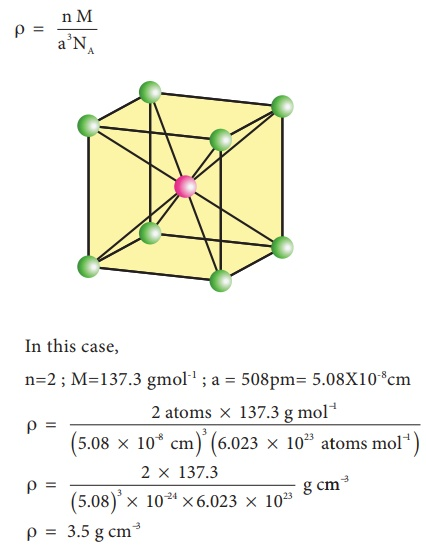Home | | Chemistry 12th Std | Calculation of unit cell dimensions and density

# Calculation of unit cell dimensions and density

Calculations involving unit cell dimensions, Calculation of density

## Calculations involving unit cell dimensions:

X-Ray diffraction analysis is the most powerful tool for the determination of crystal structure. The inter planar distance between two successive planes of atoms can be calculated using the following equation form the X-Ray diffraction data 2dsinθ = nλ

The above equation is known as Bragg’s equation.

Where

λ is the wavelength of X-ray used for diffraction.

θ is the angle of diffraction

By knowing the values of θλ and n we can calculate the value of d.

d = n λ /2sin θUsing these values the edge of the unit cell can be calculated.

## Calculation of density:

Using the edge length of a unit cell, we can calculate the density ( ρ ) of the crystal by considering a cubic unit cell as follows.Equation (6) contains four variables namely ρ , n , M and a . If any three variables are known, the fourth one can be calculated.

Example 2

Barium has a body centered cubic unit cell with a length of 508pm along an edge. What is the density of barium in g cm-3?

Solution:

In this case,

n=2 ; M=137.3 gmol-1 ; a = 508pm= 5.08X10-8cmTags : Chemistry , 12th Chemistry : UNIT 6 : Solid State
Study Material, Lecturing Notes, Assignment, Reference, Wiki description explanation, brief detail
12th Chemistry : UNIT 6 : Solid State : Calculation of unit cell dimensions and density | Chemistry